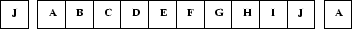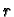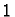Next: 2.4 Initial conditions Up: 2. Choices in the Previous: 2.2 Neighborhood size   Contents

# 2.3 Boundary conditions

In the formal definition of cellular automata, one usually requires the lattice to be infinite in all dimensions. For considerations of computability and complexity, this is reasonable and necessary. But of course it is impossible to simulate a truly infinite lattice on a computer (unless the active region always remains finite). Therefore we have to prescribe some boundary conditions. Another reason for using certain boundary conditions can be that the problem we want to simulate has certain natural boundaries.

There are three kinds of boundaries we will consider here. These are periodic, reflective, and fixed value boundaries.

Periodic boundaries are obtained by periodically extending the lattice. In Figure 2.9 we show the one-dimensional version.In practice, one needs to store only the original array and a boundary of width, whereis the radius of the neighborhood of the CA. The periodic boundary conditions come closest to simulating an infinite lattice, and are therefore often used.

The two-dimensional version of periodic boundaries, where top and bottom are connected, and the left and right edges are connected, leads to the topology of a torus (It is not possible to obtain the topology of a sphere with a regular lattice of arbitrary size). Figure 2.10 shows the example automaton with periodic boundaries. In this figure, as well as the following, we show the actual lattice surrounded by a boundary layer of width one, which in this case is a copy of one row/column from the opposite edge. No cell in this topology sees'' the boundary in any way and thus it is closest to an infinite lattice.

Applet 2.10: Example automaton with periodic boundaries. A boundary layer of width one is also shown.

Reflective boundary conditions are obtained by reflecting the lattice at the boundary. Figure 2.11 shows the situation in one dimension.This type of boundary is best suited for boundaries where the system to be simulated also has a boundary, and where the value of the physical variables is not fixed (e.g., von-Neumann, i.e., zero-flux boundary conditions in a diffusive system). Often these boundary conditions can be usefully combined with other, e.g. periodic boundary conditions on different boundaries. To simulate a long channel, one would use periodic boundaries in horizontal, and reflective boundaries in vertical direction. A two-dimensional realization of our example CA with reflective boundaries in both directions is shown in Figure 2.12.

Applet 2.12: Example automaton with reflective boundaries. A boundary layer of width one is also shown.

Fixed value boundary conditions are obtained by simply prescribing a fixed value for the cells on the boundary. This value must be determined from the application. In Figure 2.13 we show the example automaton with a value of(recovering) fixed at all boundary cells. This boundary condition corresponds to Dirichlet boundary conditions for partial differential equations.

Applet 2.13: Example automaton with constant value ofon the boundaries.

All three boundary conditions can be combined, so that different boundaries can have different conditions. Of course, if one edge has periodic boundary conditions, the opposite edge must also have periodic conditions.

Two other possible ways to treat the boundaries come to mind: One is to extend the lattice at each step so that it keeps expanding as structures in the CA touch the boundary. The disadvantage is that in many cases structures can grow quite rapidly, so that the simulation area can get too big quite quickly.

Another possibility would be to design special rules for the boundaries, where cells have fewer neighbors. The disadvantage of this approach is that there are many different types of special neighborhoods (consider the corners). A more general solution is to define a special state which is used as a constant boundary condition and does not occur inside the simulation domain. The rules can then test for the appearance of this boundary state in the neighborhood of a cell and thus introduce special behaviour with a very general mechanism. This mechanism can also be used to introduce internal boundaries.Next: 2.4 Initial conditions Up: 2. Choices in the Previous: 2.2 Neighborhood size   Contents
Jörg R. Weimar